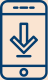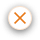#### Helping you invest with confidence# Light Up your Portfolio with SIP

18 Feb 2022 0 COMMENT## Introduction:

When you invest in a mutual fund, you want the investment process to be as easy and convenient as possible. The way you invest in a mutual fund should not only be flexible and straightforward for you, but it should also be accessible in your pockets. With Diwali approaching around the corner, your expenses might be on the higher side. From buying new clothes to entertaining guests, your costs this month might be sky-rocketing. You might not have enough to spare to invest a huge-lump sum of money in a mutual fund. But what if you could invest in a mutual fund with as little as ₹100 per month.? That is where systematic investment planning comes into place. You can invest in a mutual fund of your choice with an amount that will not cause a bigger hole in your pocket.

## How does SIP work?

With a systematic investment plan, you can invest money in a frequency that is convenient to you. The frequency you want to invest in will change from one fund house to another, but usually, you can pay either weekly, monthly, quarterly, semi-annually, or annually.

Assume you want to invest in a mutual fund. You can invest either a lump-sum payment at the beginning of a systematic investment plan at intervals. If you want to invest ₹ 20,000 in a mutual fund, instead of making this as a single payment with the SIP option, you can invest ₹500 every month for 40 months. This SIP option can be used in various mutual fund schemes.

## Calculating the right amount to invest via SIP

But how will you know the right amount to invest in a mutual fund through SIP.? You need to know the amount of money to invest to earn the returns you expect. There is a mathematical formula to calculate this amount.

Let us take an example where you want to invest ₹5000 per month for one year at a rate of interest of 12%.

M= P({1+i] n-1]/i* (1+i)

M  = Amount that you will receive on the maturity of the mutual fund

P   = amount that you will invest through SIP

n= Number of payments you have want to make

I    = rate of interest

n = 12

i =12%/12 =0.01

P = ₹5000

As per formula, the amount you will receive is calculated as

M= 5000*({1+0.01] ^{12}-1}/ 0.01) *(1+0.01)

That is a complicated and time-consuming calculation. Complex calculations done by hand might also not give you an accurate answer at times. To make this process easier, online stock brokers offer a free SIP calculator where you can find the right amount you need to start investing in a SIP within seconds.

Steps to calculate SIP using the calculator:

1)      Enter the monthly instalment, this can be any amount up to ₹25,000.

2)      Enter your expected rate of return, this can be upto 30%

3)      Enter the period, this can be up to 40 years

Using the above-mentioned Number, we can find out in an instant that your total investment amount of ₹60,000 via SIP at a 12% rate of return will give you returns of ₹4,047 taking your total value of investment from ₹60,000 to ₹64,047 in one year.

You can use the above calculator to input different values to see which would be the right amount to invest with different rates of intertest at different periods.